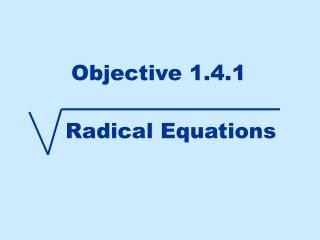DownloadDownload PresentationObjective 1.4.1

# Objective 1.4.1

Télécharger la présentation## Objective 1.4.1

- - - - - - - - - - - - - - - - - - - - - - - - - - - E N D - - - - - - - - - - - - - - - - - - - - - - - - - - -
##### Presentation Transcript

2. To solve an equation with a radical First isolate the radical + 3 + 3 This means to get any terms not under the square root on the other side of the equal sign 2 2 Now square both sides You must square the whole side NOT each term. A square "undoes" or cancels a square root Now solve for x + 1 + 1 You MUST check this answer Since you squared both sides of the equation, negatives disappear. It is possible to get an answer that doesn't work when you plug it back in It checks!

3. Let's try another one: First isolate the radical Remember that the 1/3 power means the same thing as a cube root. - 1 - 1 Now since it is a 1/3 power this means the same as a cube root so cube both sides 3 3 Now solve for x - 1 - 1 Let's check this answer It checks!

4. a solution that you find algebraically but DOES NOT make a true statement when you substitute it back into the equation. One more to see extraneous solution: The radical is already isolated 2 2 Square both sides You must square the whole side NOT each term. This must be FOILed You MUST check these answers Since you have a quadratic equation (has an x2 term) get everything on one side = 0 and see if you can factor this Doesn't work!Extraneous It checks!

5. Objective 1.4.2 Equations in Quadratic Form The "u" Substitution Method

6. Before we solve the above equation, let's solve a quadratic equation that we know how to solve. Factor Set each factor = 0 and solve Let's use this to solve the original equation by letting u = x2.

7. If u = x2 then square both sides and get u2 = x4. Substitute u and u2 for x2 and x4. Factor Set each factor = 0 and solve Now that we've solved for u we have to re-substitute to get x back. Remember u = x2 so let's substitute. Solve for x by square-rooting both sides and don't forget the 

8. You can determine if an equation is of quadratic form where you can use the "u" substitution method if you call the middle variable and power u and then square it and get the first term's variable and power. So let u = z1/4 and get u2 = z1/2. Substitute u and u2 for z1/4 and z1/2. Factor & set each factor = 0 and solve Solve for z by raising both sides to the 4th power

9. Let's try one more. Call the middle variable u and then square it to see if you get the first term's variable. So let u = x3 and get u2 = x6. Substitute u and u2 for x3 and x6. Factor & set each factor = 0 and solve Solve for x by taking the cube root of both sides